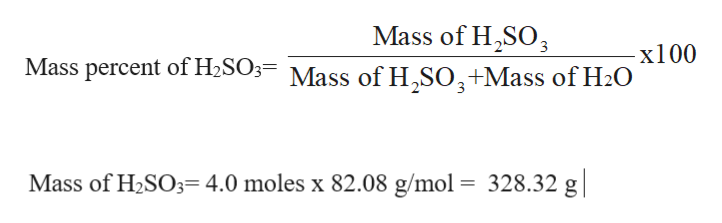# A 4.0 m H2 S O3 solution would have what mass percent of H2 S O3 in this solution? The molar mass of H2 S O3 is 82.08 grams. Just the number, please.HINT: 4.0 m H2SO3 = 4.0 mol H2 S O3/1 kg H2O and % H2 S O3 = [mass H2 S O3/(mass H2S O3 + mass H2O)] x 100%OR HINT: 4.0 m H2SO3 = 4.0 mol H2 S O3/1 kg H2O and% H2 S O3 = [mass H2 S O3 over the sum of mass H2S O3 and mass H2O)] all multiplied by 100%

Question
160 views

A 4.0 m HS O3 solution would have what mass percent of HS O3 in this solution? The molar mass of HS O3 is 82.08 grams. Just the number, please.
HINT: 4.0 m H2SO3 = 4.0 mol H2 S O3/1 kg H2O and % H2 S O3 = [mass H2 S O3/(mass H2S O3 + mass H2O)] x 100%

OR HINT: 4.0 m H2SO3 = 4.0 mol H2 S O3/1 kg H2O and

% H2 S O3 = [mass H2 S O3 over the sum of mass H2S O3 and mass H2O)] all multiplied by 100%

check_circle

Step 1

Mass percent of H2SO3 need to calculate with the help of molality of given solution.

Step 2

Molality of H2SO3 = 4.0 m = 4.0 moles / 1 kg water

Molar mass of H2SO3 = 82.08 g/mol

Mass of H2SO3= Moles x molar masshelp_outlineImage TranscriptioncloseMass of H2SO3 -x100 Mass percent of H2SO3= Mass of H,SO,+Mass of H2O Mass of H2SO34.0 moles x 82.08 g/mol = 328.32 g| fullscreen
Step 3

Mass of water = 1 kg = 1000 g

Hence total mass ...

### Want to see the full answer?

See Solution

#### Want to see this answer and more?

Solutions are written by subject experts who are available 24/7. Questions are typically answered within 1 hour.*

See Solution
*Response times may vary by subject and question.
Tagged in

### Solutions